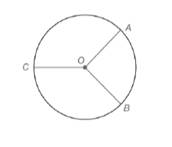Chapter 6.1, Problem 9E### Elementary Geometry for College St...

6th Edition
Daniel C. Alexander + 1 other
ISBN: 9781285195698

#### Solutions

Chapter
Section### Elementary Geometry for College St...

6th Edition
Daniel C. Alexander + 1 other
ISBN: 9781285195698
Textbook Problem
1 views

# Given: A O ¯ ⊥ O B ¯  and  O C ¯  bisects  A C B ⌢  in  ⊙ OFind: a) m A B ⌢ b) m A C B ⌢ c) m B C ⌢ d) m ∠ A O C

To determine

(a)

To Find:

If AO¯OB¯ and OC¯ bisects ACB in O find mAB.

Explanation

Given:

The provided figure is given below:

Postulate used:

Central angle postulate:

In a circle, the degree of a central angle is equal to the degree measure of its intercepted arc.

Calculation:

Since, AO¯OB¯ then mAOB=90o.

Therefore, in a circle, the degree of a central angle is equal to the degree measure of its intercepted arc

To determine

(b)

To Find:

If AO¯OB¯ and OC¯ bisects ACB in O find mACB

To determine

(c)

To Find:

If AO¯OB¯ and OC¯ bisects ACB in O find mBC.

To determine

(d)

To Find:

If AO¯OB¯ and OC¯ bisects ACB in O find mAOC.

### Still sussing out bartleby?

Check out a sample textbook solution.

See a sample solution

#### The Solution to Your Study Problems

Bartleby provides explanations to thousands of textbook problems written by our experts, many with advanced degrees!

Get Started

#### Prove that limx0x2cos(1/x2)=0.

Single Variable Calculus: Early Transcendentals, Volume I

#### Evaluate the expression sin Exercises 116. (23)2

Finite Mathematics and Applied Calculus (MindTap Course List)

#### limx0cscx= a) 0 b) c) d) does not exist

Study Guide for Stewart's Single Variable Calculus: Early Transcendentals, 8th